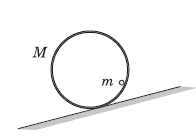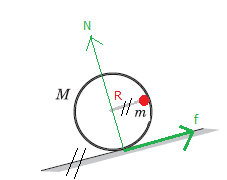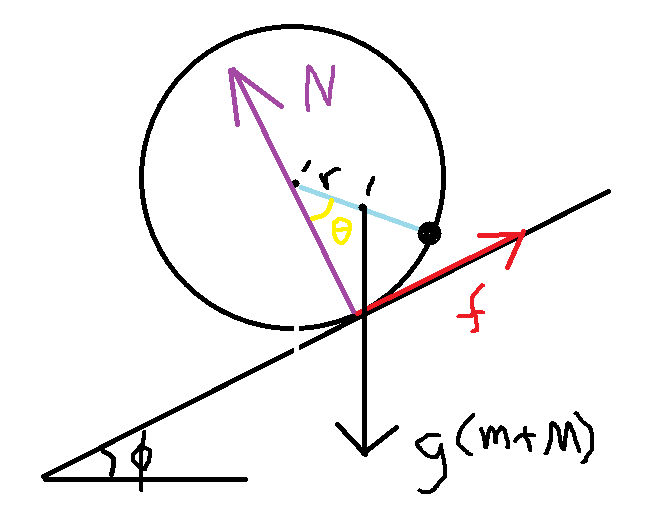# A composite body on a slope

• Rikudo
That's OK. One can find ##\mu## at some angle ##\varphi## below the horizontal line and then maximize ##\mu## with respect to ##\varphi##. Just a little bit more work, but that's what I did to explore what ##\mu## looks like as a function of ##\varphi.##f

#### Rikudo

Homework Statement
A thin rod of mass m is welded on inner surface of a thin-walled pipe of mass M parallel to the axis of the pipe. The composite body thus formed is placed on a uniform slope as in the figure. Inclination of the slope is adjustable. Find range of coefficient of friction between the pipe and the slope for which the body slides down without rotation.
Relevant Equations
TorqueHere is the hint that the book gave me:

"For the maximum value of μ, the rod must be to the extreme right i.e. horizontally rightwards of the axis of the pipe"

I think what it meant is the same as this:Note: in the calculation below, ##r## is the distance from the center of pipe to the CoM of the system and ##r = mR/(m+M)##

So, the torque calculation (with the system's CoM as origin) for maximum μ will be :
##N r = f R##
##Nr=NμR##
##m/(m+M)=μ##

But, the book says that it is ##m/\sqrt{M(M+2m)}##

Where did I do wrong?

•Delta2
torque wrt CoM: torque from normal force = Nrsinθ = torque from friction force = μN*L
Find L as an expression of r and θLast edited by a moderator:
••Delta2 and Rikudo
The weight of the rod will also produce a torque
This will be neutralized by torque produced by the pipe's weight. Hence, in the torque equation, we only use friction and normal force.

•malawi_glenn
This will be neutralized by torque produced by the pipe's weight. Hence, in the torque equation, we only use friction and normal force.
Yeah I forgot that it should only slip, not rotate at all.
Look at the pic above, try to calculate µ as a function of θ

@Rikudo now picture is correct...

AH! Now I got it. Thanks!

•malawi_glenn
"For the maximum value of μ, the rod must be to the extreme right i.e. horizontally rightwards of the axis of the pipe"

I think what it meant is the same as this:
View attachment 304258
For information, the hint says:
"For the maximum value of μ, the rod must be to the extreme right i.e. horizontally rightwards of the axis of the pipe"​

But in your diagram, the rod (red dot) is not at the extreme right, and therefore not at the same horizontal level (i.e. not at the same height) as the axis of the pipe.

•Lnewqban and malawi_glenn
But in your diagram, the rod (red dot) is not at the extreme right, and therefore not at the same horizontal level (i.e. not at the same height) as the axis of the pipe.
That's OK. One can find ##\mu## at some angle ##\varphi## below the horizontal line and then maximize ##\mu## with respect to ##\varphi##. Just a little bit more work, but that's what I did to explore what ##\mu## looks like as a function of ##\varphi.##